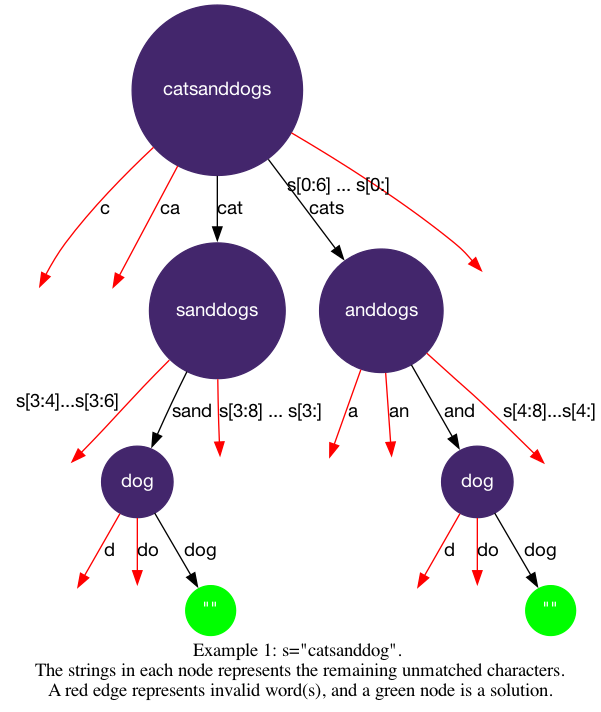# LeetCode Word Break II Solution

Given a string `s` and a dictionary of strings `wordDict`, add spaces in `s` to construct a sentence where each word is a valid dictionary word. Return all such possible sentences in any order.

Note that the same word in the dictionary may be reused multiple times in the segmentation.

Example 1:

Input: `s = "catsanddog", wordDict = ["cat","cats","and","sand","dog"]`

Output: `["cats and dog","cat sand dog"]`

Example 2:

Input: `s = "pineapplepenapple", wordDict = ["apple","pen","applepen","pine","pineapple"]`

Output: `["pine apple pen apple","pineapple pen apple","pine applepen apple"]`

Explanation: Note that you are allowed to reuse a dictionary word.

Example 3:

Input: `s = "catsandog", wordDict = ["cats","dog","sand","and","cat"]`

Output: `[]`

Constraints:

• `1 <= s.length <= 20`
• `1 <= wordDict.length <= 1000`
• `1 <= wordDict[i].length <= 10`
• `s` and `wordDict[i]` consist of only lowercase English letters.
• All the strings of `wordDict` are unique.

## Solution

We can apply the backtracking1 template to solve this problem. Fill in the logic.

• `is_leaf`: `start_index == len(s)`, when all the letters are used.
• `get_edges`: `w = s[start_index:end_index+1]` where `start_index <= end_index < len(s)`, are the possible words starting at `start_index`.
• `is_valid`: is `w in wordDict`? `w` is valid if it's in the dictionary.

#### Implementation

``````1def wordBreak(self, s: str, wordDict: List[str]) -> List[str]:
2    def dfs(start_index, path):
3        if start_index == len(s):
4            ans.append(" ".join(path))
5            return
6        for end_index in range(start_index, len(s)):
7            w = s[start_index:end_index+1]
8            if w in wordDict:
9                path.append(w)
10                dfs(end_index+1, path)
11                path.pop()
12    ans = []
13    dfs(0, [])
14    return ans``````